## Saturday, March 31, 2012

Blog Entry #67

Introduction

The goal is to find the velocity of a ball, and the angle it travels, given the initial velocity and angle.

The next two tables are for reference. Thanks to my cousin Gina for helping me with the Coefficient of Restitution! You are the best!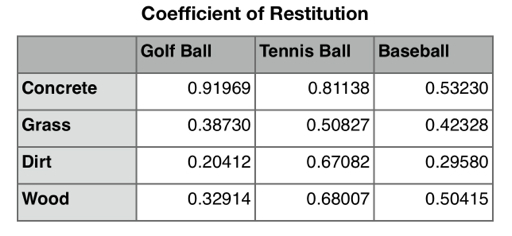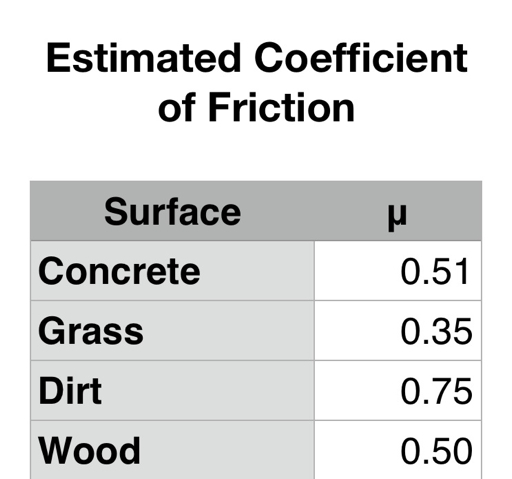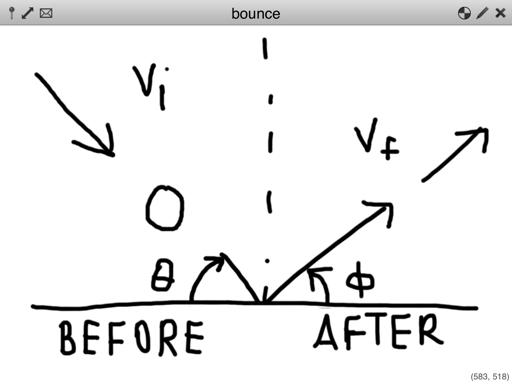Variables:

Given:
v_i = initial velocity
θ = angle of impact before the bounce

Want to find:
v_f = final velocity
Φ = angle of travel after the ball bounces

Assumptions:
1. Gravity has negligible impact. We are talking about the moment just before the ball bounces to just after the ball bounces.
2. The coefficient of resistution (COR) is measured experimentally (see table). I thank my awesome cousin Gina Ramirez helped me with getting data. (see table above)
3. The x-direction is "forwards/backwards" and the y-direction is "up towards the sky/down towards the earth"
4. The coefficeint of friction only affects the y-direction (is this correct?)
5. Kinetic energy is not conserved.
6. The ground does not move and maintains a zero velocity.

Variables:
COR = coefficient of restitution, depending on the type of ball and surface the ball is being bounced on
μ = coefficient of friction, dependent on the surface the ball is being bounced on
v_ix, v_iy = initial velocity of the ball in the x-direction and y-direction, respectively
v_fx, v_fy = final velocity of the ball in the x-direction and y-direction, respectively

COR = √ ( (height of ball after the bounce) / (height of the ball before the bounce) )

Since momentum must be conserved in both the x and y directions:

m * v_i * cos θ = m * v_f * cos Φ
m * v_i * sin θ = m * v_f * sin Φ

m * v_i * cos θ - m * v_f * cos Φ = 0
m * v_i * sin θ - m * v_f * sin Φ = 0

m * v_i * cos θ - m * v_f * cos Φ = m * v_i * sin θ - m * v_f * sin Φ

m * ( v_i * cos θ - v_f * cos Φ ) = m * ( v_i * sin θ - v_f * sin Φ )

Note the definition of Impulse, I = m * Δv = Δp

Section A: Coefficient for Restitution

COR = v_fy / v_iy

v_fy = v_iy * COR

Hence: v_fy - v_iy = v_iy * COR - v_iy = v_iy * (COR - 1)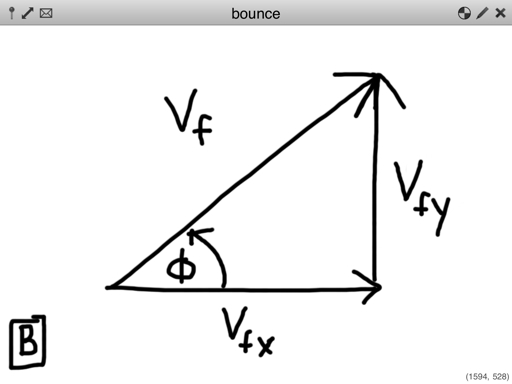Section B: Angle After Bounce

tan Φ = v_fy / v_fx
tan Φ = (v_iy * COR) / v_fx
cot Φ = v_fx / (v_iy * COR)
COR * cot Φ = v_fx / v_iy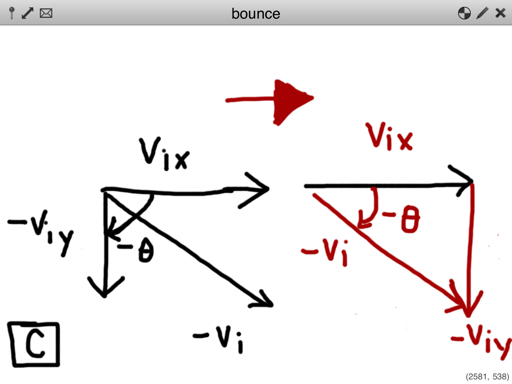Section C: Angle Before Bounce

tan (-θ) = -v_iy / v_ix
- tan θ = - v_iy / v_ix
tan θ = v_iy / v_ix

Main:

Finding Final Angle After Bounce

Impulse, taking friction ( μ ) into consideration:

m * μ * (v_fy - v_iy) = m * (v_fx - v_ix)
μ * (v_fy - v_fi) = v_fx - v_ix

From Section A:

μ * v_iy * (COR - 1) = v_fx - v_ix
μ * (COR - 1) = v_fx/v_iy - v_ix/v_iy

From Section B:

μ * (COR - 1) = COR * cot Φ - v_ix/v_iy

From Section C:

μ * (COR - 1) = COR * cot Φ - tan θ
μ * (COR - 1) + tan θ = COR * cot Φ
(μ * (COR - 1) + tan θ) / COR = cot Φ
COR / (μ * (COR - 1) + tan θ) = tan Φ

which implies that:

Φ = arctan ( COR / (μ * (COR - 1) + tan θ) )

Finding Final Velocity

From Section B:

v_fx = (v_iy * COR) / tan Φ

We also know that:

cos Φ = v_fx / v_f

Then:

v_f = v_fx / cos Φ
v_f = (v_iy * COR) / (tan Φ * cos Φ)
v_f = (v_iy * COR) / sin Φ
v_f = (v_i * sin θ * COR) / sin Φ

Results

Final Angle:

Φ = arctan ( COR / (μ * (COR - 1) + tan θ) )

Final Velcoity:

v_f = (v_i * sin θ * COR) / sin Φ

Sources:

"Friction" http://physics.info/friction Retrieved 3/25/2012

"Coefficient of Restitution" Wikipedia. http://en.wikipedia.org/wiki/Coefficient_of_restitution Retrieved 3/22/2012

H. Brody "That's how the ball bounces" from "The Physics of Sports" edited by Armenti Angelo. American Institute of Physics: New York. 1992

This blog is property of Edward Shore. © 2012

## Monday, March 26, 2012

### HP 50g: Elastic Collisions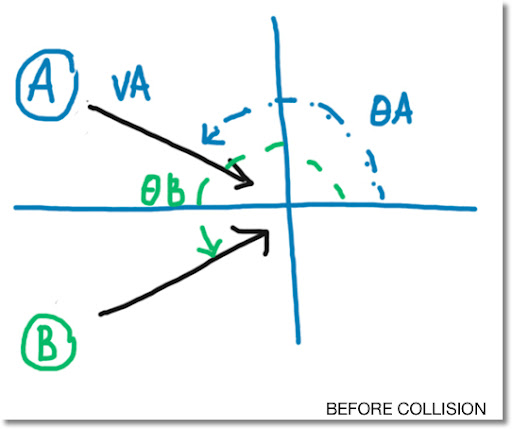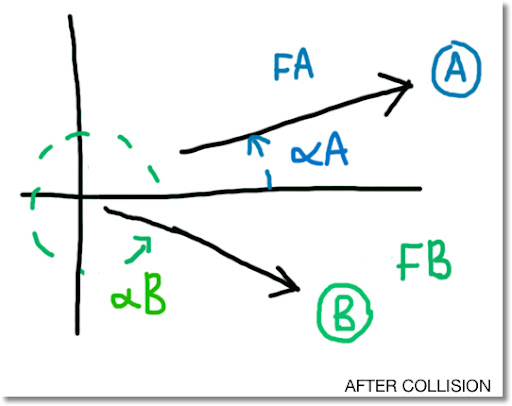Welcome to Blog Entry #66

This is a program for some solving elastic collision problems for the Hewlett Packard 50g calculator.

Variables (refer to the diagrams above):

MA = mass of object A
VA = initial velocity of object A
θA = initial angle of A

MB = mass of object B
VB = initial velocity of object B
θB = initial angle of B

FA = final velocity of object A
αA = final angle of object A

FB = final velocity of object B
αB = final angle of object B

To type θ, press ΑLPHA right-shift T

To type α, press ALPHA right-shift A

Program:

PROGRAM NAME: ELASTIC

<< DEG
{ ' MA * VA * COS(θA) + MB * VB * COS(θB) = MA * FA * COS(αA) + MB * FB * COS(αB) '
' MA * VA * SIN(θA) + MB * VB * SIN(θB) = MA * FA * SIN(αA) + MB * FB * SIN(αB) '
' .5 * MA * VA^2 + .5 * MB * VB^2 = .5 * MA * FA^2 + .5 * MB * FB^2 ' }
'EQ' STO
MINIT
"Elastic Collisions"
{ ' MA ' ' VA ' ' θA ' ' MB ' ' VB ' ' θB ' ' FA ' ' αA ' ' FB ' ' αB ' }
MITM MSOLVR >>

Angle Guide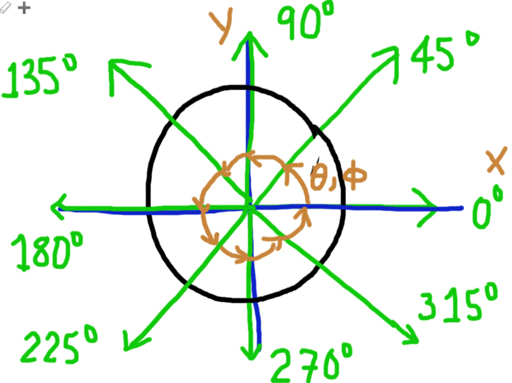Examples: (FIX 4)

Example 1:

Known:

MA = 1.2450
VA = 40.0000
θA = 30.0000

MB = 0.9780
VB = 45.0000
θB = 225.000

FA = 25.0000
αA = 170.0000

Solved:

FB = 57.1505
αB = 40.2485

Example 2:

Known:

MA = 4.0000
VA = 3.4565
θA = 0.0000

MB = 4.0000
VB = 4.5000
θB = 270.0000

FA = 2.8750
αA = 170.0000
αB = 80.0000

Solved:

FB = 10.2957

This blog is property of Edward Shore. © 2012

## Wednesday, March 21, 2012

### Collisions: Elastic and Inelastic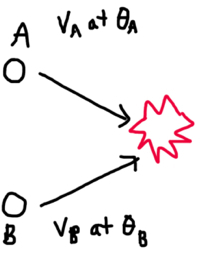Welcome to blog entry #65! This is the first blog entry using the new Apple iPad (3rd generation, white with 64 GB, so excited)

Introduction

In physics when two objects collide, in general, one of two things can happen:

* They can stick together, producing an inelastic collision, or

* They can bounce off of each other, producing an elastic collision.

In realty, each collision lies somewhere in between inelastic and perfectly elastic, but we will deal with the general cases here.

As the diagram above shows objects A and B are headed on a collision course; each one with their own mass, velocity, and direction. If A and B combine, let C represent the object formed from both A and B.

Define the following variables as:

MA = mass of object A
MB = mass of object B
MC = combined mass of objects A and B = mass of object C

Prior to Collision
VA = initial velocity of object A
VB = initial velocity of object B
θA = initial angle of direction of object A
θB = initial angle of direction of object B

After Collision - Inelastic Collision
FC = final velocity of the aggregate object after objects A and B collide
ΦC = final angle of the aggregate object after objects A and B collide

After Collision - Elastic Collision
FA = final velocity of object A
FB = final velocity of object B
ΦA = final angle of object A
ΦB = final angle of object B

In this blog entry, we will assume we know all the initial conditions VA, VB, θA, and θB. Additionally, assume that friction is negligible, and not a factor in calculation.

Angle is used to dictate the direction of the object. 0° is considered going due right (east) (postitive in the x-direction only). If the direction angle is 90°, the object is going due up (north), positive in the y direction only. Refer to the diagram below.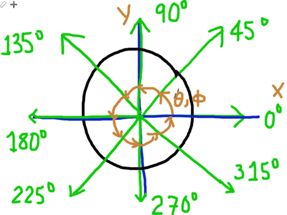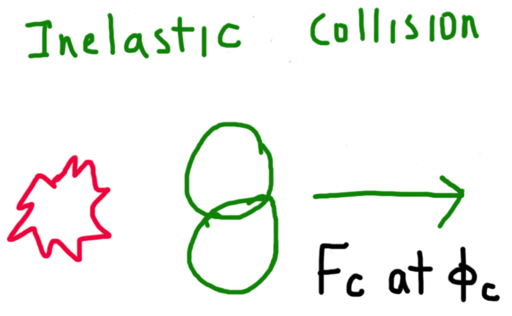Inelastic Collisions

In inelastic collisions, when objects collide, they tend to "stick" together. Examples include a car wreck or a when football (rugby) defensive end tackles a wide receiver.

Only momentum is conserved in inelastic collisions. Kinetic energy that was given by the two objects is lost. Some energy is either vibrations or heat given by the combined object.

The equation for momentum is: P = m * v, where m is mass and v is velocity.

When solving for the general case, we have to deal with the total momentum in both the x-direction and the y-direction.

The initial momentum for each direction respectively is:

Px = MA * VA * cos θA + MB * VB * cos θB (1)
Py = MA* VA *sin θA + MB * VB * sin θB (2)

Since momentum is conserved, the total initial momentum must be equal to the total final momentum, in the x- and y-direction. Hence:

Px = MC * FC * cos ΦC (3)
Py = MC * FC * sin ΦC (4)

where MC = MA + MB (5)

As a result, we have two equations of two unknowns: VC and ΦC. A calculator (such as HP 50G, TI 89, TI nSpire) or a sufficient mathematics computer program can solve the set of equations easily.

Since the equations are relatively simple, we can find explicit solutions by the following strategy.

First, solve for ΦC. Either equation (3) or (4) can be used. For this blog entry, I will use equation (3).

Px = MC * FC * cos ΦC
Px / (MC * FC) = cos ΦC

Then ΦC = arccos (Px / (MC * FC)) (6)

Sub into (4) to get:

Py = MC * FC * sin (arccos (Px / (MC * FC)))

Note that sin(arccos x) = √ (1 - x^2). Also cos(arcsin x) = √ (1 - x^2)

Then:

Py = MC * FC * √ ( 1 - (Px / (MC * FC))^2 )
Py = MC * FC * √ ( ( MC^2 FC^2 - Px^2 ) / (MC^2 * FC^2) )
Py = (MC * FC * √ ( MC^2 FC^2 - Px^2 ) ) / (MC * FC)
Py = √ ( MC^2 * FC^2 - Px^2 )

Square both sides and rearrange terms:

MC^2 * FC^2 = Py^2 + Px^2

Solving for FC gives:

FC = √ ( (Py^2 + Px^2) / MC^2 ) (7)

(We can just take the principal root, since the ΦC will take care of the direction of object C)

In Summary:
MC = MA + MB
FC = √ ( (Py^2 + Px^2) / MC^2 )
ΦC = arccos (Px / (MC * FC))

This is a solution for an inelastic collision for any two objects of any mass, velocity, and direction angle.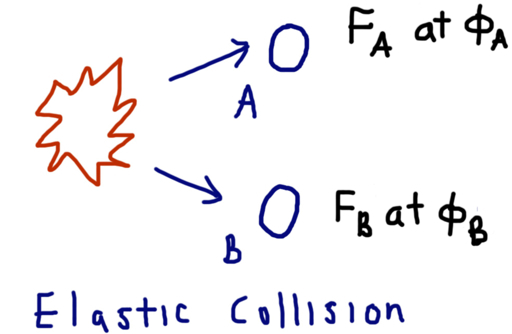Elastic Collisions

In perfectly elastic collisions, when objects collide, they tend to "bounce off" each other. Examples include a sub-atomic gas particle collision or two billiard balls hitting each other.

Like inelastic collisions, momentum is conserved in inelastic collisions. Unlike inelastic collisions, kinetic energy is also conserved.

The equation for momentum is: P = m * v, where m is mass and v is velocity.

When solving for the general case, we have to deal with the total momentum in both the x-direction and the y-direction.

The initial momentum for each direction respectively is:

Px = MA * VA * cos θA + MB * VB * cos θB (1)
Py = MA* VA *sin θA + MB * VB * sin θB (2)

The equation for kinetic energy is: KE = 1/2 * m * v^2. Kinetic energy does not take separate compenent directions into account.

The initial kinetic energy is:

KE = 1/2 * MA * VA^2 + 1/2 * MB * VB^2 (8)

Since momentum and kinetic energy are conserved, the total initial momentum must be equal to the total final momentum, in the x- and y-direction; and the total initial kinetic energy must be equal to the total final kinetic energy. Hence:

Px = MA * FA * cos ΦA + MB * FB * cos ΦB (9)
Py = MA * FA * sin ΦA + MB * FB * sin ΦB (10)
KE = 1/2 * MA * FA^2 + 1/2 * MB * FB^2 (11)

Note if we do not know anything about the final conditions, then we are dealing with a system of three equations with four unknowns: FA, ΦA, FB, and ΦB.

In order for this system to be solvable, we must know one of the final conditions. Then the system becomes three equations and three unknowns. A calculator (such as HP 50G, TI 89, TI nSpire) or mathematics computer program can assist in solving the set of equations.

What if one of the velocities is known?

Let's assume that FA is known. We can simplify the system of equations (9), (10), and (11). A similar strategy can be used if FB is known.

KE = 1/2 * MA * FA^2 + 1/2 * MB * FB^2
1/2 * MB * FB^2 = KE - 1/2 * MA * FA^2
FB^2 = 2 * KE / MB - MA * FA^2 / MB

Then:

FB = √ ( ( 2 * KE - MA * FA^2 ) / MB ) (12)

Now we are left with the system of two equations with two unknowns: ΦA and ΦB. Use your favorite calculating tool to solve for them.

Px = MA * FA * cos ΦA + MB * FB * cos ΦB (9)
Py = MA * FA * sin ΦA + MB * FB * sin ΦB (10)

For more information of elastic and inelastic collisions, click here (Hyperphysics Page). . Thanks Mime Grigsby, a very good friend of mine and fellow mathematician.

Until next time,

Eddie

Diagrams: drawn using Whiteboard

This blog entry is dedicated to Uncle Ralph (born March 21). Me and my family miss you and love you. Thank you for touching our lives.

This blog entry is property of Edward Shore. © 2012

## Saturday, March 17, 2012

### Billiards - Determining Final Velocity (Program for TI 84+ and HP 50g too!)

Welcome to blog entry #64!

HAPPY ST PATRICK'S DAY (WEEKEND)!

Billiards and Math

Ever wondered what happens with you take the cue stick, strike the cue ball, hit your target ball and the target ball sails right into a pocket? If you played billiards, commonly known as pool, you have experienced this many times.

We can use mathematics and physics to describe what happens when the cue ball hits the target ball. Since the balls do not "stick" together like steamed rice, the collision is considered to be nearly elastic.

In an elastic collision, two properties are conserved: momentum and kinetic energy. Momentum is the product of mass and it's velocity. Kinetic energy is the energy of movement. The equations for momentum and kinetic energy are, respectively:

P = m * v

KE = 1/2 * m * v^2

Note that direction is not considered in calculating kinetic energy.

Problem:

You hit a cue ball at a certain velocity VI, and hit a target ball at angle θ. We want to find out the direction of the cue ball after collision and the velocity of both balls.

Assume the following:

1. The masses of the cue ball and the target ball are the same. This will allow a great simplicafication in calculation, as we will see.

2. The Intial direction of the ball is 0° (due right). As a visual, you are on the left hitting the ball to due right.

Note the diagrams below: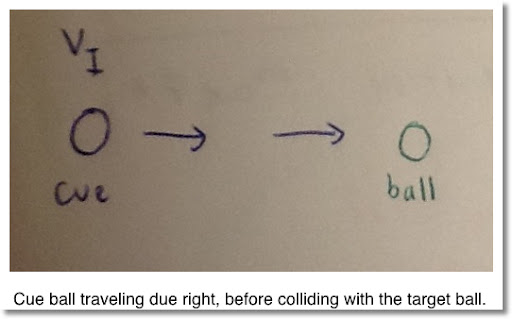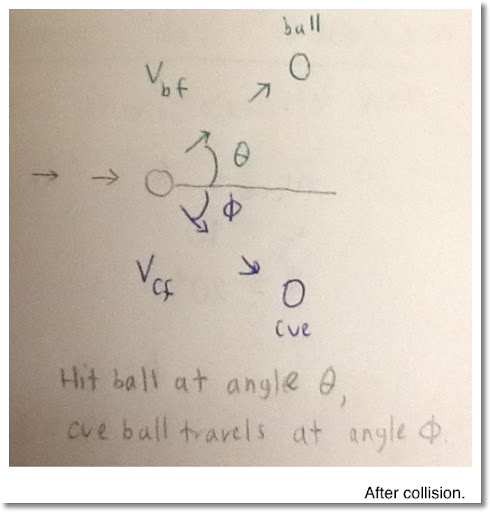3. The angles created after the hit add up to 90°.

From the diagram above, θ + Φ = 90°.

4. The cue ball does not hit any balls other than the target ball. If more than two balls were involved, calculation can get hairy, quick.

5. The cue ball is hit at it's sweet spot, which eliminates any friction developing between the ball and the felt of the pool table. According to The Real World Physics Problems web site, the sweet spot is 1.4 times the ball's radius.

An American billiard ball has a diameter of about 2.25 in, or 5.715 cm. The ball has a typical weight of 5.5 to 6 oz, or 156 to 170 grams. This link has more information on billiard balls.

Solution, Assuming All Masses Are Equal

Let:

VI = initial velocity of the cue ball, going due right. In the x-direction, VI cos 0° = VI. In the y-direction, VI sin 0° = 0, as expected.

VCF = final velocity of the cue ball

VBF = final velocity of the target ball. Note since the target ball was at rest, it's initial velocity is zero.

Φ = Angle where the cue ball goes after collision. Simply put, Φ = 90° - θ.

MC = mass of the cue ball

MB = mass of the target ball

Note: We assumed the masses were equal, MB = MC.

Momentum and kinetic energy has to be preserved. So for momentum in the x-direction:

MC * VI = MB * VBF cos θ + MC * VCF cos Φ

and momentum in the y-direction:

0 = MB * VBF sin θ - MC * VCF sin Φ

Note: Φ is a angle in the clockwise direction and sin (-Φ) = -sin Φ

and for kinetic energy:

1/2 * MC * VI^2 = 1/2 * MB * VBF^2 + 1/2 * MC * VCF^2

Note: Since mass is the same for all balls (MC = MB), mass "cancels" out in all three equations.

To Recap:
VI = VBF cos θ + VCF cos Φ
0 = VBF sin θ - VCF sin Φ
1/2 * VI^2 = 1/2 * VBF^2 + 1/2 * VCF^2

Making use of the trigonometric identities:

sin (90° - x) = cos x
cos (90° - x) = sin x

The equations simplify to:
VI = VBF cos θ + VCF sin θ
0 = VBF sin θ - VCF cos θ
1/2 * VI^2 = 1/2 * VBF^2 + 1/2 * VCF^2

Observe that if we solve for VBF in the second equation, we get:
VBF = VCF * (cos θ / sin θ)

Then:
VI = VBF cos θ + VCF sin θ
VI = VCF * (cos θ / sin θ) *cos θ + VCF sin θ
VI = VCF * (cos^2 θ / sin θ ) + VCF sin θ
VI = (VCF * cos^2 θ + VCF sin^2 θ) / sin θ

Note: sin^2 x + cos^2 x = 1 for an angle x.

And:
VI = VCF / sin θ

Solving for VCF yields:
VCF = VI sin θ

Let's get VBF in terms of VI:
VBF = VCF * (cos θ / sin θ)
VBF = VI sin θ* (cos θ / sin θ)
VBF = VI cos θ

Before we can celebrate, we need to satisfy the conservation of kinetic energy, not just momentum.
1/2 * VI^2 = 1/2 * VBF^2 + 1/2 * VCF^2

First, let's just multiply everything by 2:
VI^2 = VBF^2 + VCF^2
VI^2 = (VI cos θ )^2 + (VI sin θ)^2
VI^2 = VI^2 * (cos^2 θ + sin^2 θ )
VI = VI

So this checks out because we assumed the masses of both the cue ball and target ball were equal. .

Final solution - All Masses being Equal:

VBF = VI cos θ
VCF = VI sin θ
Φ = 90° - θ

What if the Mass of the Cue Ball and Target Ball are not equal?

Let:

MC = mass of the cue ball

MB = mass of the target ball

If I use the strategy of solving for VCF and VBF above, I get:

VCF = VI * sin θ.
VBF = (MC / MB) * VI * cos θ

We are working with elastic collisions, so conservation of kinetic energy must be considered:

1/2 * MC * VI^2 = 1/2 * MB * VBF^2 + 1/2 * MC * VCF^2

But:
1/2 * MB * VBF^2 + 1/2 * MC * VCF^2
= 1/2 * (MB * (VI * cos θ * MC / MB)^2 + MC * VI^2 * sin^2 θ))
= 1/2 * MC * VI^2 * (cos^2 θ / MB + sin^2 θ)
≠ 1/2 * MC * VI^2

Yep. No go. Confession, I thought the above way was good to go when MC ≠ MB too.

Here is a real solution if MC ≠ MB:

VBF = (MC * VCF) / (MB * tan θ)

And we have to solve the quadratic equation for VCF:

0 = VCF^2 * (MB * MC^2 * sin θ + MC^2 * cos^2 θ)
+ VCF * (MB * MC)
+ (MB * sin θ * (MC * VI + MC * VI^2))

The following calculator programs solve for Φ, VCF, and VBF with the assumption that the mass of both the cue ball and target ball are the same.

Variables for the programs:
C = VI
A = θ

TI 84 Plus:

NAME: BILLIARD

Degree
Disp "Deg MODE"
Input "INIT. VEL.",C
Input "ANGLE HIT",A
C*SIN(A) → S
C*COS(A) → T
Disp "ANGLE CUE=", 90 - A
Pause
Disp "FINAL VEL. CUE=",S
Disp "FINAL VEL. BALL=",T

HP 50g:

NAME: BILLIARD

<< DEG
"Degrees Mode" MSGBOX
"Initial Velocity, Cue" PROMPT
"Hit Angle" PROMPT
→ C A
<< "Finals:"
90 A - →NUM "Angle, Cue" →TAG
C A SIN * →NUM "Vel., Cue" →TAG
C A COS * →NUM "Vel., Ball" →TAG
>> >>

Test data:
VI = 5, θ = 15°; Results: VCF ≈ 1.2941, VBF ≈ 4.8296

VI = 2, θ = 30°; Results: VCF = 1, VBF = √3 ≈ 1.7321

Thank you, hope you enjoyed this blog entry. Next time I probably be blogging on a third generation iPad. For the first 64 entries, I have been using the Blogger+ App by ThinkTek Co. Ltd. on my iPad 2. Thanks everyone!

Eddie

This blog is property of Edward Shore. © 2012

## Thursday, March 15, 2012

### Projectiles - A Realistic Point of View (Linear Drag)

Projectile Motion

This blog is about projectile motion. Most resources give an introductory treatment of projectile motion. And yes, I will present the non-air resistance model in this section. But I am also going to present a more realistic model, taking into account air resistance, size of the projectile, and temperature.

All amounts are presented in SI (kilograms, meters, seconds) units.

Quick Conversions
1 ft = 3.280839895 m
1 lb = 0.4535924 kg

Simple Projectile Motion

A perfect projectile can be described by the following equations:

x(t) = v0 t cos θ
y(t) = v0 t sin θ - 0.5 g t^2

Where
v0 = initial velocity
θ = Intial angle, in degrees
g = gravitational acceleration constant = 9.80665 m/s^2 = 32.17404856 ft/s^2
And we assume the starting height is zero (on the ground)

Note that air resistence, features of the object, and other outside objects are ignored.

Example: Hitting a Golf Ball

Use the simplistic model to plot a trajectory with an initial velocity of 150 mph (67.056 m/s) at a 35° angle.

The trajectory lasts for approximately 7.844 seconds, with a range of approximately 430.86 meters and a height of approximately 75.4 meters.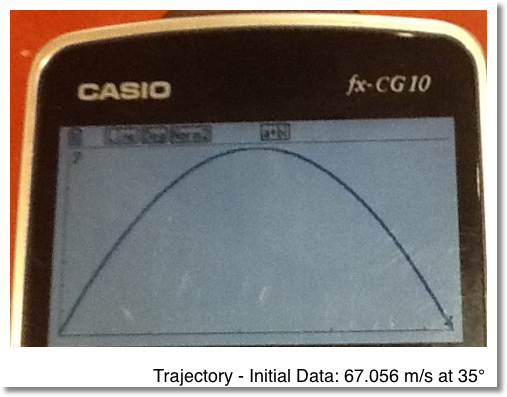A More Realisitc View of Projectile Motion

The equations above describe simple projectile motion. However, if we want a realistic picture, we have to consider factors such as temperature, air pressure, and the size of the projectile.

So of the variables we will consider are the drag coefficient, the density of air, and the object's terminal velocity.

Note: Linear drag is assumed. This is best for objects moving at slow speeds. No lift is used.

Drag Coefficient

The drag coefficient is a dimensionless quantity that quantifies the resistance of an objects's movement, taking into consideration factors such as the object's size, shape, and smoothness.

Approximate Drag Coefficients:
Perfect Smooth Baseball: 0.1
Baseball: 0.3
Golf Ball: 0.4
Tennis Ball: 0.6

Density of Air

The density of air can be determined using the Ideal Gas Law:

P V = N R T

where
P = absolute pressure = 101325 kPa
R = Specific Gas Constant = 287.058 J/kg K
T = temperature in Kelvins
N = number of moles (mass)
V = volume of the gas
ρ = air density = N/V in kg/m^3

Solving for ρ gives:

ρ = P / (R T) ≈ 352.9774471/T

Note: To convert degrees Celsius to Kevlins, add 273.15.

Terminal Velocity

When an object falls, it reaches a terminal velocity when the drag becomes equal to the object's weight. At terminal velocity, the object no longer accelerates.

Terminal Velocity is measured by:

V = √ ( ( 2 × mass of object × g ) / ( drag coefficient × ρ × surface area of object ) )

TROJECT
Calculator: CASIO fx-CG10 (Prizm)

Note: Let [triangle] be the symbol for the "stop and display triangle"

{.3, .6, .4} → List "C"
{.145, .057, .045} → List "M"
{.041043, .003318, .001385} → List "S"
Deg
ClrText
"Init Velocity="?→ V
"Init Angle="? → θ
9.80665 → G
Lbl 1: 1 → I : Goto Y
Lbl 2 : 2 → I : Goto Y
Lbl 3 : 3 → I : Goto Y
Lbl Y
"86°F/30°C",C,"77°F/25°C",D,
"68°F/20°C",E,"59°F/15°C",F,
"50°F/10°C",G,"41°F/5°C",H,
"32°F/0°C",I
Lbl A : 1.1839 → P : Goto Z
Lbl B : 1.2041 → P : Goto Z
Lbl C : 1.2250 → P : Goto Z
Lbl D : 1.2466 → P : Goto Z
Lbl E : 1.2690 → P : Goto Z
Lbl F : 1.2920 → P : Goto Z
Lbl G : 1.3163 → P : Goto Z
Lbl H : 1.3413 → P : Goto Z
Lbl I : 1.3943 → P : Goto Z
Lbl Z
List "M"[I] → M
√ (( 2 × M × G) ÷ (List "C"[I] × P × List "S"[I])) → W
M × G ÷ W → K
Solve(-MGX ÷ K + M ÷ K × ( 1 - e^(-KX ÷ M)),100) → E
MV cos θ ÷ K × ( 1 - e^(-KE ÷ M)) → R
"Range="
R [triangle]
-M ÷ K × ln ((MG) ÷ K × (V sin θ + MG ÷ K)^-1) → F
-MGF ÷ K + M ÷ K × (V sin θ + MG ÷ K) × (1 - e^(-KF ÷ M)) → H
"Height="
H [triangle]
ClrGraph
ViewWindow -.5, R + .5, 1, -1, H+1, 1, 0, E, .05
θ → A
ParamType
G SelOff
G SelOn 1
"MV cos A ÷ K × (1 - e^(-KT ÷ M))" → Xt1
"-MGT ÷ K + M ÷ K × (V sin A + MG ÷ K) × (1 - e^(-KT ÷ M))" → Yt1
DrawGraph

-----------

Variables:

M = mass of object, in grams
V = initial velocity, in metes/seconds
A = θ = initial angle, in degrees
C = drag coefficient
S = radius of the object
P = gas density
K = terminal velocity

Revisiting the Example: The Golf Ball

Use the proposed model to plot a trajectory with an initial velocity of 150 mph (67.056 m/s) at a 35° angle. Assume it is 77°F outside (25°C).

The trajectory has a range of approximately 163.46 meters and a height of approximately 45.02 meters. It lasts about 6.177 seconds.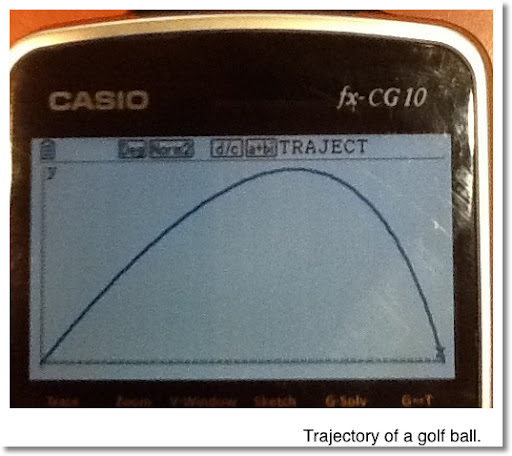References:

Article: Erlichson, Herman. "Maximum Projectile range with drag and lift, with particular application to golf." The College of Staten Island, 1982

From:

Armenti, Angelo Jr. (editor) The Physics of Sports American Institute of Physics 1983 pg. 71-78

Terminal Velocity (NASA.gov)

Drag Coefficient (NASA.gov)

Drag on a Baseball (NASA.gov)

Air Pressure

## Wednesday, March 14, 2012

### My Favorite Number: π

Why π is My Favorite Number

The number π describes a beautiful relationship between a circle's circumference, area, and radius.

The π is irrational, which means the decimal expansion goes without end.

π ≈ 3.14159265358979323846... (this is from memory). Mathematicians from ancient Greece on forward have worked on obtaining more digits of π. I think the current count is a trillion decimal places.

The gamma of 1/2 is the square root of π.

π is involved in the normal distribution function: f(x) = e^(-2x)/√ (2 π)

Unlike pie, enjoying π doesn't make anyone fat. Furthermore, unlike pie, π has no calories.

The (basic) sine and cosine functions have a period of 2 π radians. (360°)

The number π is involved in infinite series. For example:
π /4 = 1 - 1/3 + 1/5 - 1/7 + 1/9 - 1/11 + 1/13 - 1/15 + 1/17 - 1/19 + 1/21...

or π = 4 Σ ((-1)^n / (2n + 1), n = 0 to infinity)

This series converges slowly - at 10,000 terms I only get 3 decimal points (3.14169264367).

I was born on March 14 - and π = 3.14 to two decimal places.

## Tuesday, March 6, 2012

### Spirals

This blog will about spirals. Why? I thought it would be fun. And I like spirals.

Regular Spirals

Source: http://www.mathematische-basteleien.de/spiral.htm

Equations:
Rectangular
x^2 + y^2 = a^2 (arctan y/x)^2

Polar
r = a θ

Parametric
x = a t cos t
y = a t sin t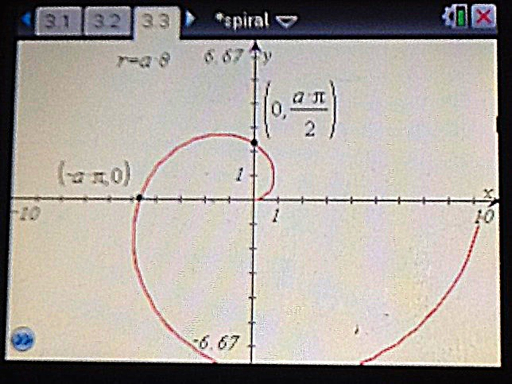Finding the y-intercept and x-intercept, as shown above, of a spiral, assuming 0 ≤ θ ≤ 2 π

We can use the rectangular equation to find these points.

x^2 + y^2 = a^2 (arctan y/x)^2

Y-Intercept - Point (0, y)

y^2 = a^2 (arctan y/0)^2

Note:
1. y/0 is undefined
2. tan (π /2) is undefined. So is tan (n π / 2) where n is an integer.

Use π / 2 as a solution.

Then:

y^2 = a^2 * (π / 2)^2

Take the square root of both sides, since y>0, use the positive root.

y = a * π / 2

The y-intercept is (0, a * π / 2)

X-Intercept - Point (x, 0)

x^2 = a^2 (arctan 0)^2

Note tan(n π.)=0 where n is an integer. Let's use π. (n = 1)

x^2 = a^2 π^2

Since the x-intercept lies left of 0, select the negative square roof. Therefore:

x = -a π.

The x-intercept is (-a π, 0).

Area of a Spiral (0 ≤ θ ≤ 2 π)

Use the polar equation: r = a θ

Using the general polar integral:

A = ∫ (1/2 * r^2 d θ , lower limit, upper limit)

A = ∫ (1/2 * a^2 * θ^2 d θ , 0, 2 π)
= a^2 / 2 * (8 π^3 / 3 - 0)
= 4 a^2 π^3 / 3

Slope of a Spiral

Polar Form:

∂r/∂θ = a

Parametric Form:

∂x/∂t = ∂/∂t (a * t * cos t)
= ∂/∂t (a * t) * cos t + a * t * ∂/∂t (cos t)
= a * cos t - a * t * sin t
= a * (cos t - t * sin t)

∂y/∂t = ∂/∂t (a * t * sin t)
= ∂/∂t (a * t) * sin t + a * t * ∂/∂t (sin t)
= a * sin t + a * t * cos t
= a * (sin t + t * cos t)

Stretch Spirals

What if we can "stretch" spirals? We can if alter the parameters of the parametric form:

x(t) = a * t * cos t
y(t) = b * t * sin t

Where a ≠ b.

Two graphical examples are shown below.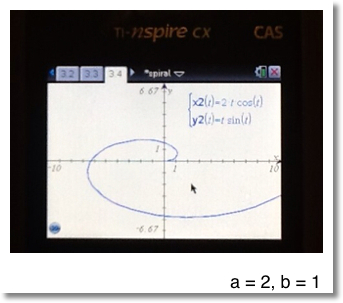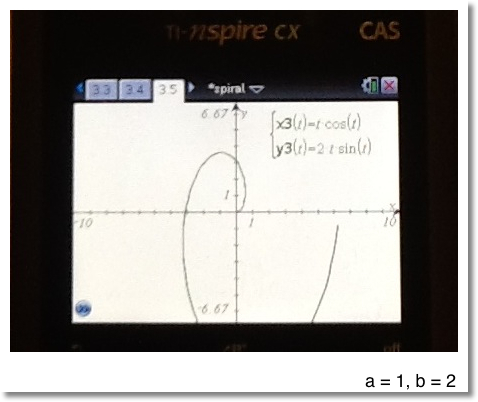Finding the Intercepts of a Stretched Spiral

I will assume that both a ≠ 0 and b ≠ 0.

Y-Intercept: Point (0, y)

0 = a * t * cos t
y = b * t * sin t

Working with the first equation:

0 = a * t * cos t
0 = t * cos t,

which implies that either t = 0 or cos t = 0

We know that cos (n π / 2) = 0 where n is an integer. Choose t = π / 2.

Then y = b * π / 2 * sin(π / 2) = b * π / 2

Then the y-intercept is (0, π / 2).

X-Intercept: Point (x, 0)

x = a * t * cos t
0 = b * t * sin t

Working with the second equation:
0 = b * t * sin t
0 = t * sin t

which implies that t = 0 or sin t = 0. We know that sin(n π) = 0 where n is an integer, let's choose n = 1 and then t = π.

Hence x = a * π * cos π = -a * π.

The x-intercept is (-a * π, 0)

Area of a Stretched Spiral

We can find the area using the following:

∫ y dx = ∫ y(t) d[x(t)]

Note that:
1. y(t) = b * t * sin t
2. d[x(t)] = a * (cos t - t * sin t) dt
3. y(t) d[x(t)] = a * b * (t * sin t * cos t - t^2 * sin^2 t)

Let Φ = (t * sin t * cos t - t^2 * sin^2 t)

The integration is split into two intervals: [0, π] and [π, 2 π]. Due to the direction of the spiral, the limits are switched.

Then the area is:
A = a * b * ( ∫ (Φ dt, π , 0 ) + ∫ ( Φ dt, 2 π , π ) )
(via Hewlett Packard HP 50g and TI nSpire CX CAS)
= a * b * (π^3 / 6 + 7 * π^3 / 6)
= a * b * (4 π / 3)

Slope of a Stretched Spiral

The derivation is similar to the slope of a regular spiral.

∂x / ∂t = a * ( cos t - t * sin t )
∂y / ∂t = b * ( sin t + t * cos t )

Until next time, Eddie.

This blog is property of Edward Shore. (c) 2012

## Thursday, March 1, 2012

### A Diary of Plots

These are some graphs I posted on both my Twitter and Facebook accounts recently. Enjoy!

Range for Bézier curves: 0 ≤ t ≤ 1

Eddie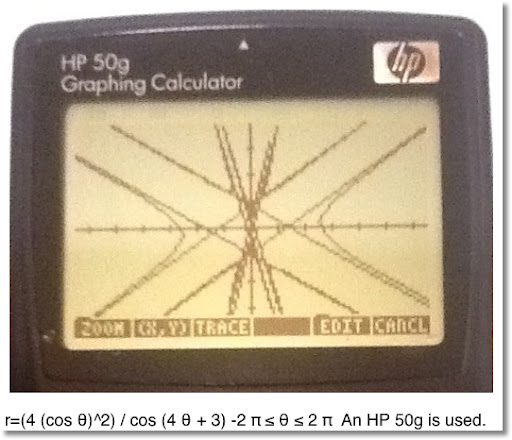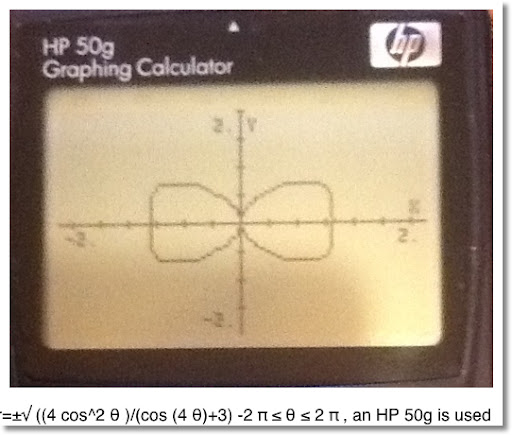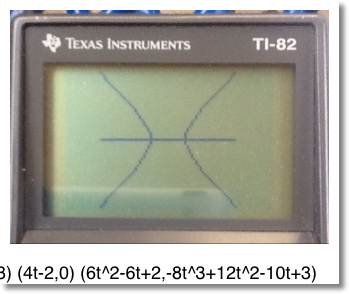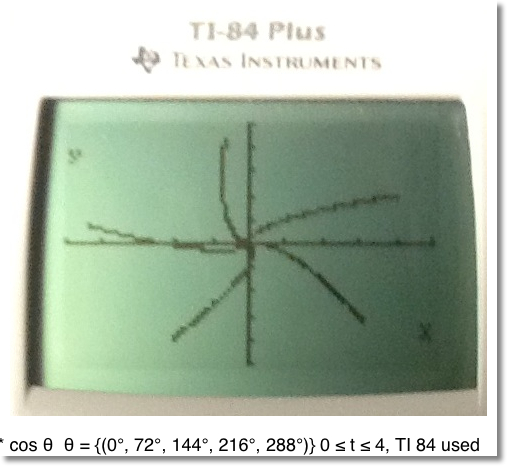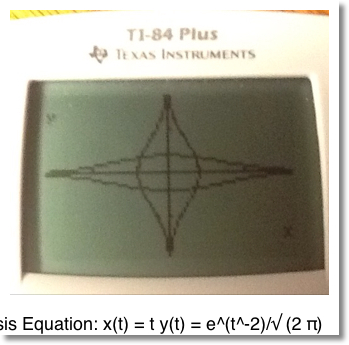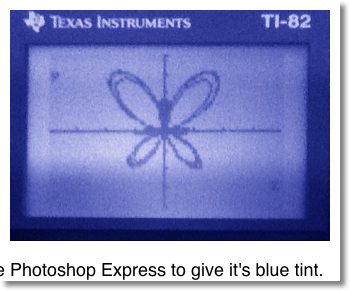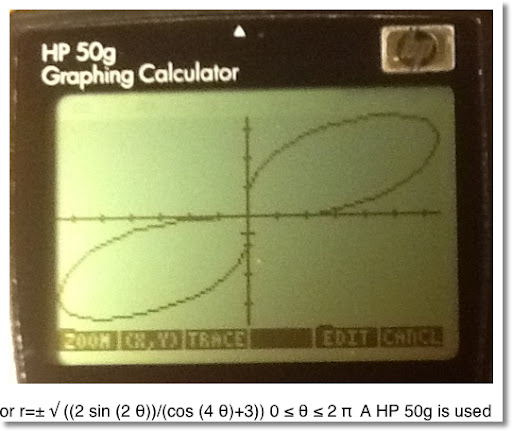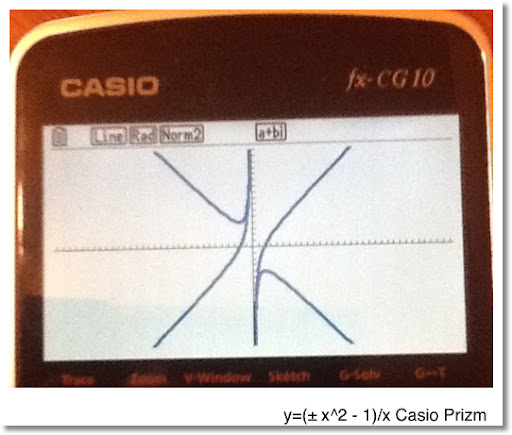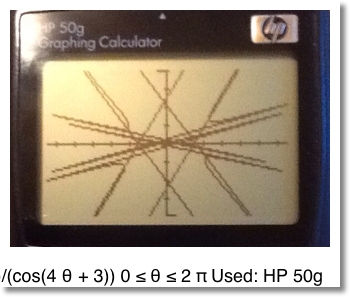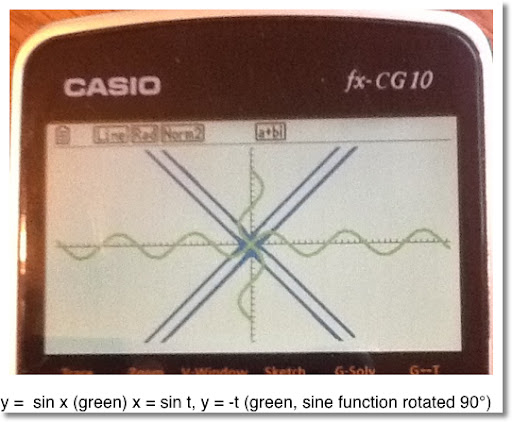### HP 15C: Error Function and Lower Tail Normal Cumulative Function

HP 15C:   Error Function and Lower Tail Normal Cumulative Function Formulas Used  Error Function  erf(x) = 2 ÷ √π * ∫( e^(-t^2) dt, t = 0 t...